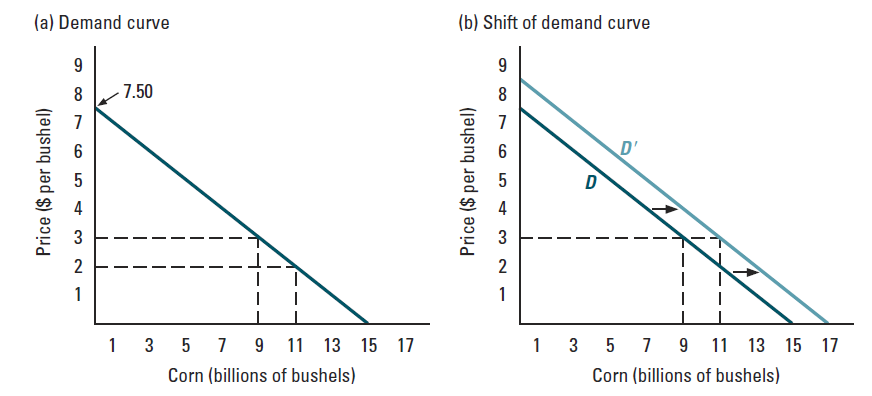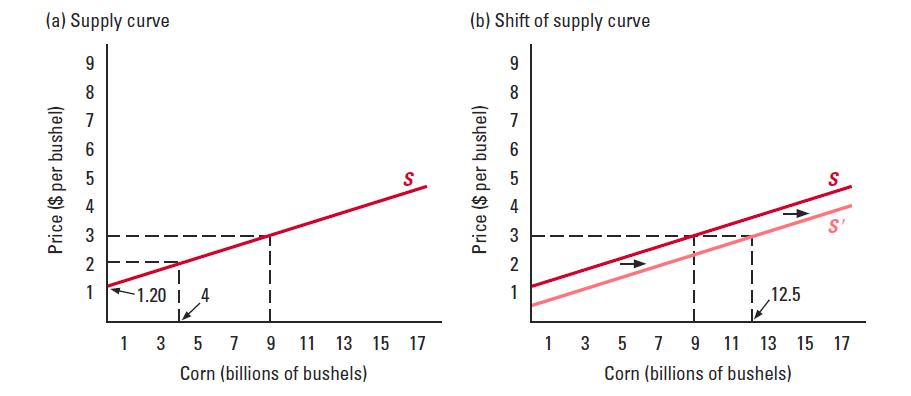# Economics Tutor near London and online

## Economics tutors near London and available online. Work with best economics tutors from LSE, Kings, UCL.

#### Understanding the demand curveFigure (a) shows a downward sloping demand curve exhibiting the law of demand, which is the negative relationship between price and quantity demanded. A movement along the demand curve is caused by a change in price, and leads to a change in quantity demanded. Additionally, a change in price is called an endogenous change.

Figure (b) shows a shift in the demand curve itself. The curve shifts because any of the exogenous changes, or determinants of demand. These factors are other than price of the good and are external “exogenous”. Namely, common determinants of demand are (i) price of other goods (ii) income of consumers (iii) number of buyers (iv) expectations of buyers (v) fashion, taste, habits. Moreover, these factors shift the entire demand curve without a change in price.

Understanding the difference between a change in quantity demanded and a change in demand is critical to understanding demand curves. This concept is tested rigorously at GCE, IB, and undergraduate level. Our economics tutors in and near London offer specialized exam preparation in-person and online economics tutoring. Undergraduate economics tutors near London are hired from LSE, Kings, UCL.

#### Understanding the supply curveFigure (a) shows the upward sloping supply curve. As price increases, quantity supplied is increased. Accordingly, this relationship shows the law of supply.
Figure (b) shows a shift in the supply curve. This depends on determinants or exogenous factors of supply. Namely, these factors are (i) cost of production (ii) expectations of price (iii) number of buyers and (iv) technology. These factors shift the entire supply curve.

#### Example

• Market demand curves derived by summing across consumers. Industry supply curves derived by summing across producers.

‘Equilibrium’ –(from Latin): ‘ equal’–‘ This encapsulates two central principles:
1. Equality : forces moving in one direction are exactly cancelled by forces moving in the other.
2. Stability : things are stationary, no longer in flux.

### Type of Problems Economists seek to answer

What will happen if we increase VAT?
How will commodity prices react to changes in exchange rates?
Will unemployment be affected by a national living wage?
Can we increase use of solar power via subsidies?
Will public spending kick start the economy?
All of the above are comparative statics questions.
“What will be the new equlibrium”

Other popular courses:

Get Started

## See the #1 economics mentoring platform in action

Request a demo